Ladder frame scaffolding of Snappy Scaffold Frames,Also known as  Walk Through Frame, Ladder Frame, Box Frame,Narrow Frame, Heavy Duty Scaffolding Shoring Frame with Frame System Components of Scaffolding Braces, Coupling Pins, Guard Rails, Goosers, Screw Jacks, U Head, Guard Rail Post.

VERTICAL FRAME (WITH PIN)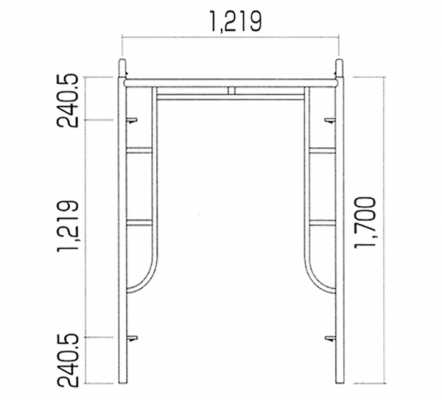W=1219  H=1700  18kg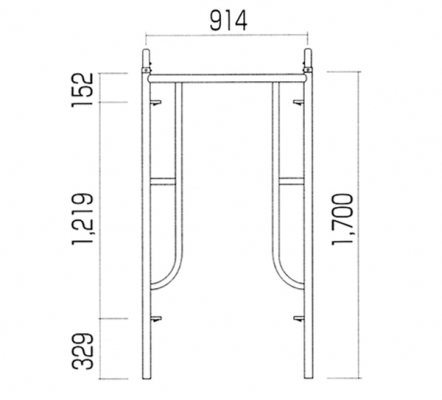W=914  H=1700  15kg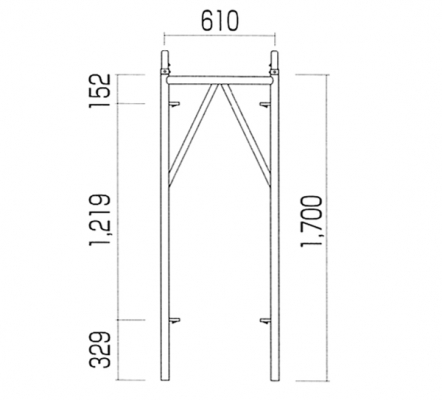W=610  H=1700  11.1kg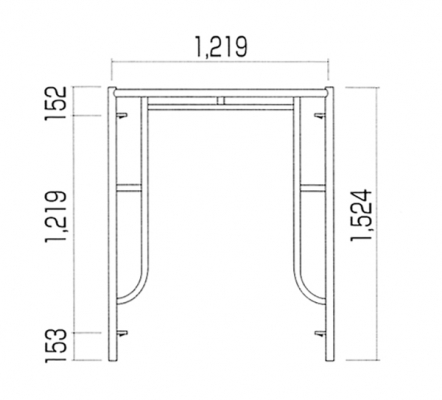W=1219  H=1524  17kg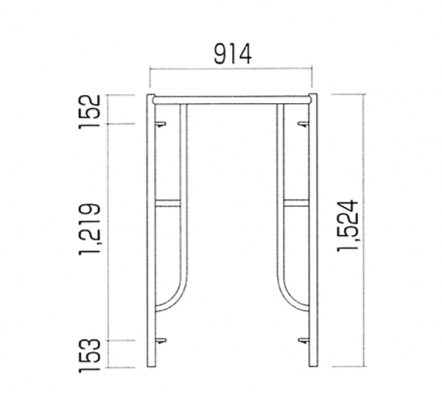W=914  H=1524  14kg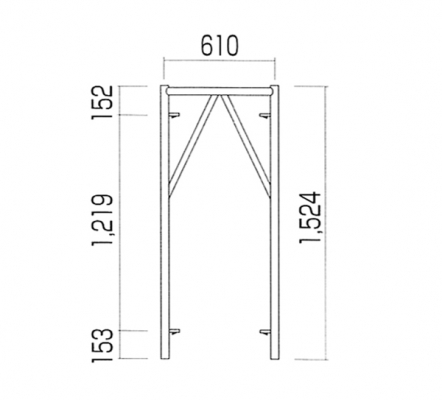W=610  H=1524 13kg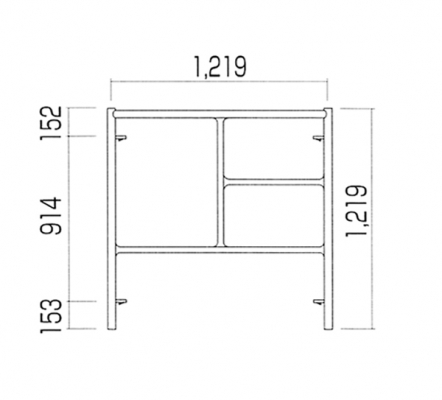W=1219  H=1219  14.5g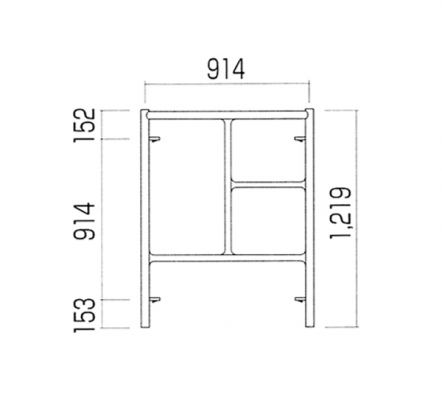W=914  H=1219 12.6kg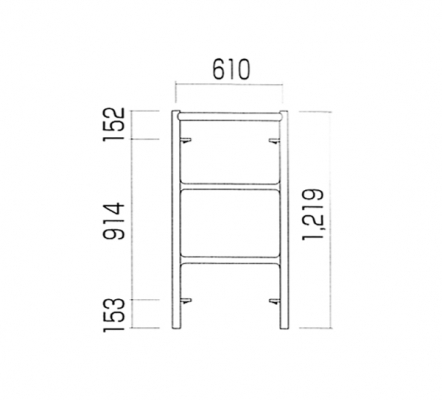W=610  H=1219 11kg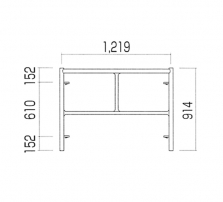W=1219  H=914  11.6kg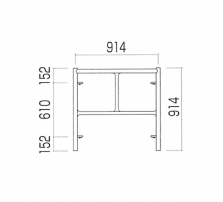W=914  H=914  9.8kg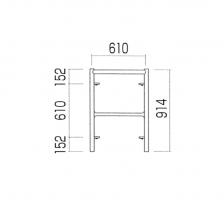W=610  H=914  10kg